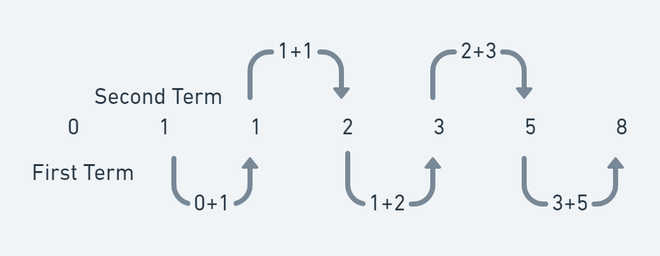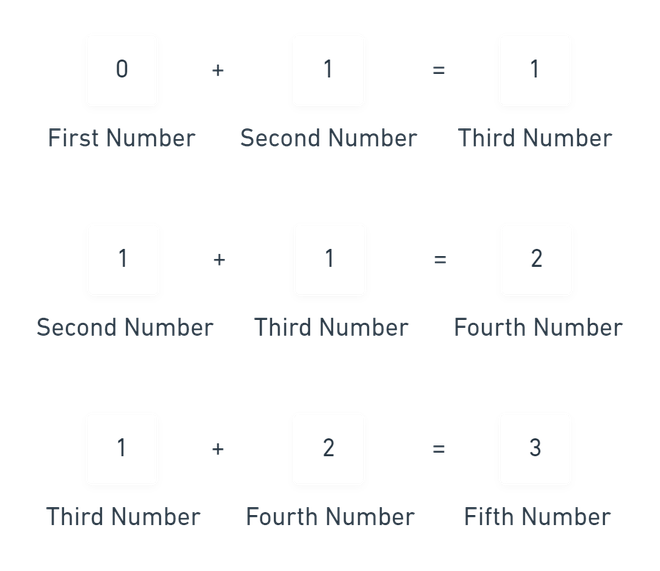Open in App
Not now

# Fibonacci Sequence Formula

• Last Updated : 29 Nov, 2022

Fibonacci sequence is one of the most known formulas in number theory. In the Fibonacci sequence, each number in the series is calculated by adding the two numbers before it. Generally, the first two terms of the Fibonacci series are 0 and 1. Fibonacci sequence was known in India hundreds of years before Leonardo Pisano Bigollo know about it. November 23rd is celebrated as Fibonacci Day, as it has the digits “1, 1, 2, 3” which is part of the sequence.

The Fibonacci sequence is as follows:

0, 1, 1, 2, 3, 5, 8, 13, 21, 34, 55, 89, 144, 233, 377, 610, 987, 1597, 2584, 4181, 6765, 10946,….

Fibonacci’s sequence is useful for its operations in advanced mathematics and statistics, computer science, economics, and nature.Fibonacci Series

Formula of Fibonacci Number

Fn = Fn-1 + Fn-2

• Fn is term number “n”
• Fn−1 is the previous term (n−1)
• Fn−2 is the term before that (n−2)Calculation of Fibonacci numbers

To calculate the 5th Fibonacci number, add the 4th and 3rd Fibonacci numbers.

### Golden Ratio

On choosing any two consecutive (one after the other) Fibonacci numbers, their ratio is near to 1.618034 and it is called Golden Ratio. It is denoted by “φ”. The golden ratio is generally can be seen in nature, and when applied in a design, it fosters natural-seeming works that are pleasing to the eye. There are numerous operations of the golden ratio in the field of architecture. For illustration, the Great Pyramid of Egypt and the Great Mosque of Kairouan is many of the architectural miracles in which the notion of the golden ratio has been applied.

For example:

Note: Golden Ratio can be calculated from Any Fibonacci sequence, it does not necessarily have to start with 2 and 3.

Calculation of Fibonacci number using Golden Ratio

Any Fibonacci number can be calculated by using this formula,

xn =  (φn − (1−φ)n)/√5

xn denotes Fibonacci number to be calculated

φ is Golden ratio that is 1.618034

For example: If you want to calculate the 7th term:

x7 = ((1.618034)7-(1-1.618034)7)/√5

x7 = 13.0000007

x7 = 13(rounded off)

The next Fibonacci number can also be calculated using Golden Ratio. Multiplying a Fibonacci number with a golden ratio will give the next Fibonacci number of the sequence. But that only works for numbers greater than 1.

Example: 13*1.618034 = 21.034442 = 21(rounded off)

### Some Problems based on Golden Ratio

Question 1: Calculate the 9th Fibonacci number if given golden ratio is 1.618034.

Solution:

We can calculate the 9th Fibonacci number by using the formula:

xn =  (φn − (1−φ)n)/√5

x9 = ((1.618034)9-(1-1.618034)9)/√5

x9 = (76.0131604-(-0.0131556197))/√5 = 34.0000021

x9 = 34

Question 2: Find the next Fibonacci number of answers calculated in the above question.

Solution:

Next Fibonacci number of 34 can be easily found by multiplying it by the Golden ratio that is 1.618034.

x10 = 34×1.61803 = 55.01302

x10 = 55(rounded off)

### Some Problems based on Fibonacci Numbers

Question 1: If the 5th and 6th terms of a Fibonacci sequence are 3 and 5 respectively, find the 7th term of the sequence.

Solution:

With the use of the Fibonacci Sequence formula, we can easily calculate the 7th term of the Fibonacci sequence which is the sum of the 5th and 6th terms.

seventh term = 5th term + 6th term

= 3+5

= 8

The 7th term of the Fibonacci sequence is 8.

Question 2: The first 4 numbers in the Fibonacci sequence are given as 1,1,2,3.

(a) What is the eighth term of the Fibonacci sequence?

(b) What is the eleventh term of the Fibonacci sequence?

Solution:

By the use of the Fibonacci number formula, we can calculate the rest of the Fibonacci numbers like 1, 1, 2, 3, 5, 8, 13, 21, 34, 55, 89.

(a) Therefore, the 8th term will be 21.

(b) 11th term will be 89.

Question 3: Find the next 3 terms for each of the following Fibonacci-style sequences.

(a) x, 4x, 5x, 9x,…

(b) 3a, 3a+b, 6a+b, 9a+2b….

Solution:

With the use of the Fibonacci Sequence formula, we can easily calculate the rest of the terms

(a) Fifth term = 5x+9x = 14x,

Sixth term = 9x+14x = 23x,

Seventh term = 14x+23x = 37x

(b) Fifth term = 6a+b+9a+2b = 15a+3b,

Sixth term = 9a+2b+15a+3b = 24a+5b,

Seventh term = 15a+3b+24a+5b = 39a+8b

Question 4: John wants to generate a Fibonacci series with the first term as 3 and the second term as 4.

(a) Find the 3rd and 4th terms.

(b) He thinks that the sum of the first ten terms is equal to eleven times the seventh term of his sequence. Check if he is correct.

Solution:

Using the 3 and 4 as first and second terms, we can calculate the rest of the terms by simply adding the last two terms.

(a) First term = 3,

Second term = 4,

Third Term = 3+4=7,

Forth term = 4+7 = 11

(b) On calculating the first ten terms of the series: 3,4,7,11,18,29,47,76,123,199.

Sum of first ten terms = 3+4+7+11+18+29+47+76+123+199 = 517

7th term = 47

Eleven times the 7th term = 11*47 = 517

As we can see that the sum of the first ten terms is equal to eleven times the seventh term of his sequence. Therefore, John was correct.

Question 5: What is the first three-digit square number that appears on the list of Fibonacci numbers, if the first 4 terms are 0,1,1,2.

Solution:

With the use of the Fibonacci Sequence formula, we can easily calculate the rest of the terms:

0,1,1,2,3,5,8,13,21,34,55,89,144,233,377,…

As we can see the first three-digit number which is a square that appears on the list of Fibonacci numbers is 144(square of 12).

My Personal Notes arrow_drop_up